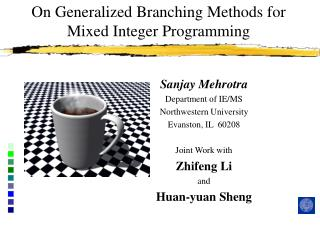Download PresentationOn Generalized Branching Methods for Mixed Integer Programming

# On Generalized Branching Methods for Mixed Integer Programming - PowerPoint PPT PresentationDownload Presentation## On Generalized Branching Methods for Mixed Integer Programming

- - - - - - - - - - - - - - - - - - - - - - - - - - - E N D - - - - - - - - - - - - - - - - - - - - - - - - - - -
##### Presentation Transcript

1. On Generalized Branching Methods for Mixed Integer Programming Sanjay Mehrotra Department of IE/MS Northwestern University Evanston, IL 60208 Joint Work with Zhifeng Li and Huan-yuan Sheng

2. Organization • Introduction and Review • Adjoint Lattice • Theoretical Results • Computational Results • Conclusions

3. Problem Formulation

4. Approaches to Branching • Branching Strategies • Branching on variables • Most infeasible branching • Strong branching • Nested Cluster Branching • Pseudo-cost branching • Branching on constraints • Branching on variables may not be efficient for solving general MIP.

5. An Example

6. Matter of Definitions

7. Example (continued)

8. An Example (continued)

9. Lenstra’s Algorithm

10. Lenstra’s Algorithm (continued)

11. Lenstra’s Algorithm (Geometry) Step 2: Step 3:

12. Pervious Work • Lenstra  proposed algorithm branching on hyperplane • Grötschel, Lovász and Schrijver  used ellipsoidal approximation directly. • Lovász and Scarf  developed Generalized Basis Reduction (GBR) algorithm that does not require ellipsoidal approximation and works with the original model, however, assumes full dimensionality. • Cook, et. al.  implemented GBR algorithm for some hard network design problems. • Wang  implemented GBR algorithm for LP and NLP (<100 integer variables). • Aardal, Hurkens, and Lenstra [98,00], Aardal et. al. , Aardal and Lenstra , proposed a reformulation technique and solved some hard equality constrained integer knapsack and market split problems using this reformulation. • Owen and Mehrotra  computationally showed that good branching disjunctions are available using heuristics for smaller problems in the MIPLIB testset. • Gao and Zhang  implemented Lenstra's algorithm and tested an interior point algorithm for finding the maximum volume ellipsoid.

13. Issues • Dimension reduction… hmm… • Don’t know what to do when continuous variables are present • Continuous variables are “projected” out • How to do this for the more general convex problem? • It is just nice to work in the original space! • Basis Reduction at every node… • Finding a feasible solution or • finding good branching directions • Ellipsoidal Approximation • We should use the modern technologies! • Feasibility problem vs. Optimization Problem • Disjunctive branching (instead of branching on hyperplanes)

15. Branching Hyperplane finding Problem

16. Ellipsoidal Approximation • John  showed the existence of k-rounding for a given convex set with non-empty interior point. • Lenstra  gave a constructive procedure for finding an ellipsoidal rounding. • Interior-Point Methods: Analytic center, Vaidya Center, Volumetric center and corresponding ellipsoidal rounding.

17. The Quality of Approximation

18. Bound on Minimum Width Using Ellipsoidal Approximation

19. How to Solve Minimum Width Problems

20. Lovasz and Scarf Basis

21. An interpretation of the Aardal, Hurkens and Lenstra Reformulation

22. Mixed Integer Programming • Feasibility Mixed Integer Linear Program (FMILP) is to

23. Mixed Integer Problem

24. Generalized B&B Method IMPACT (Integer Mathematical Programming Advanced Computational Tool) implementation of GBB. • A growing search tree

25. Knapsack Test Problems

26. Numerical Results on Hard Knapsack Problems

27. Market Split Problems

28. Larger Market Split Problems

29. # of LLL iterations and GBB Tree Size

30. Developed general branching methods for Linear and Convex Mixed Integer Programs. It is possible to develop stable codes for Dense Difficult Market Split problems using Adjoint Lattice Basis. Conclusions